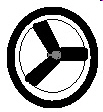3.G.2..-Test1B Partition shapes into parts with equal areas.
 Name:    3.G.2..-Test1B Partition shapes into parts with equal areas.

Multiple Choice
Identify the choice that best completes the statement or answers the question.

1.

If a shape was divided into 8 equal parts, what fraction would each of those parts be?
 a. 1/8 c. 4/8 b. 8/1 d. 8/8

2.

If a shape was divided into 19 equal parts, what fraction would each of those parts be?
 a. 19 c. 1/19 b. 1 d. 19/1

3.

If a shape was divided into 64 equal parts, what fraction would each of those parts be?
 a. 64/64 c. 64/1 b. 64/1 d. 1/64

4.

If a shape was divided into 9 equal parts, what fraction would each of those parts be?
 a. 1/9 c. 9/1 b. 9/9 d. 1

5.

If a shape was divided into 21 equal parts, what fraction would each of those parts be?
 a. 21/1 c. 11/21 b. 1/21 d. 21/21

6.

If a shape was divided into 5 equal parts, what fraction would each of those parts be?
 a. 1/5 c. 5/1 b. 5/5 d. 2/5

7.

If you tear a piece of paper into 16 equal parts, what fraction would each of those parts be?
 a. 16/16 c. 8/16 b. 16/1 d. 1/16

8.

Andy took two pieces of paper and ripped them in half.  He them put those pieces together and ripped them into equal parts again.  What fraction did each of those pieces equal?
 a. 8/1 c. 4/8 b. 1/8 d. 8/8

9.

A bicycle tire has three spokes.  What fraction would each of the parts of the wheel be?a. 3/1 c. 1/3 b. 3/3 d. 1

10.

If a shape was divided into 45 equal parts, what fraction would each of those parts be?
 a. 1/45 c. 45/45 b. 1/1 d. 45/1